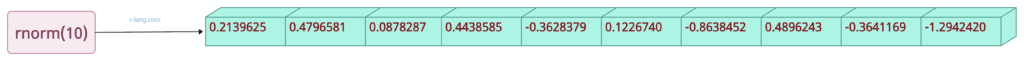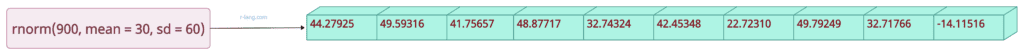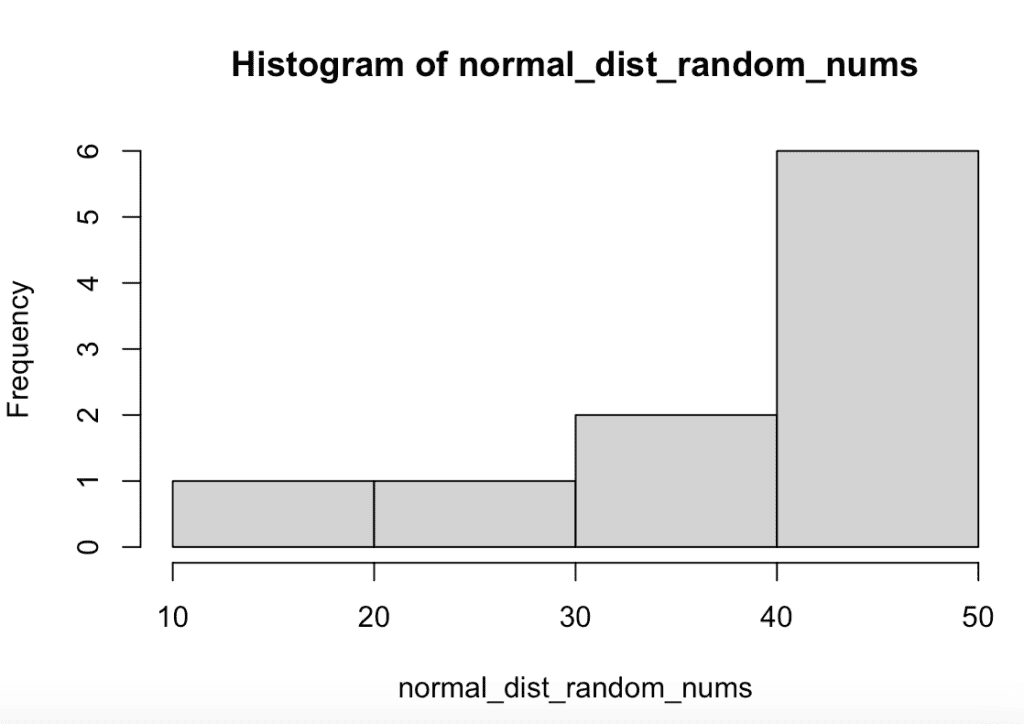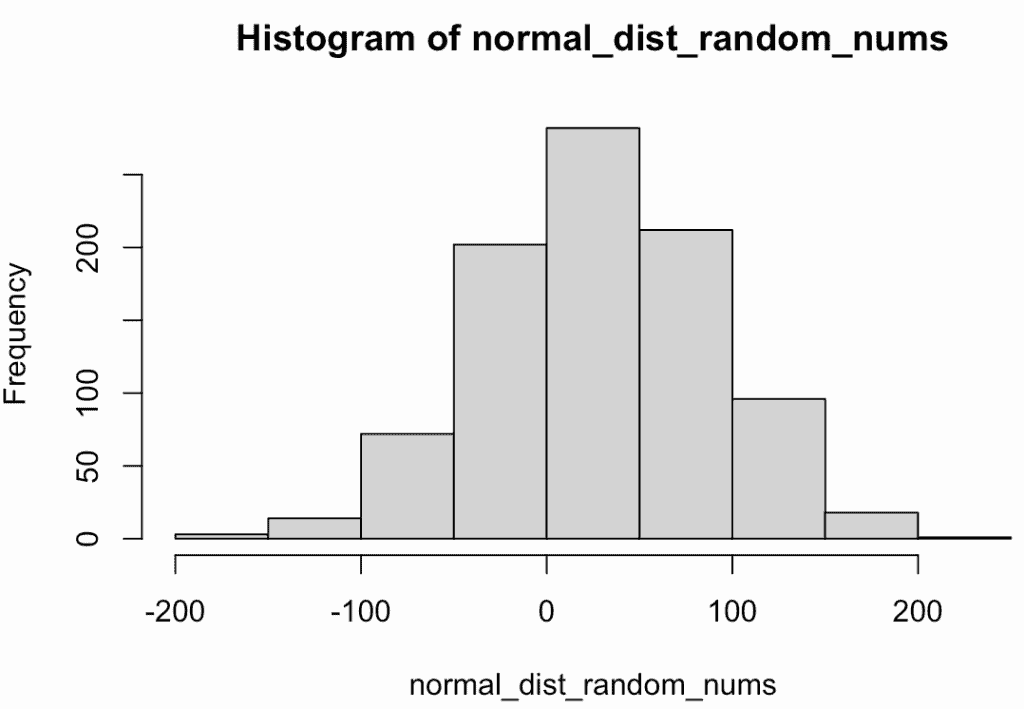# How to Generate Normal Distribution Random Numbers in R

To generate random numbers from a normal distribution, use the “rnorm()” function. It generates random numbers from a normal, continuous probability distribution with a bell-shaped curve.

## Example 1``````set.seed(99)

normal_dist_random_nums <- rnorm(10)

print(normal_dist_random_nums)``````

Output

``````  0.2139625 0.4796581 0.0878287 0.4438585 -0.3628379 0.1226740
 -0.8638452 0.4896243 -0.3641169 -1.2942420``````

## Example 2

In the function, mean, and sd are optional arguments you can specify based on your requirements.

Use the following code to generate 10 random numbers from a normal distribution with a mean of 40 and a standard deviation of 20.``````set.seed(99)

normal_dist_random_nums <- rnorm(900, mean = 30, sd = 60)
hist(normal_dist_random_nums)``````

Output

`````` 44.27925 49.59316 41.75657 48.87717 32.74324 42.45348 22.72310 49.79249
 32.71766 14.11516``````

## Example 3

To create a histogram, use the hist() function and provide the generated random numbers.

``````set.seed(99)

normal_dist_random_nums <- rnorm(10, mean = 40, sd = 20)
hist(normal_dist_random_nums)``````

OutputI have used RStudio to show the plot using the hist() function.

Let’s see another example.

``````set.seed(99)

normal_dist_random_nums <- rnorm(900, mean = 30, sd = 60)

hist(normal_dist_random_nums)``````

OutputThat’s it.